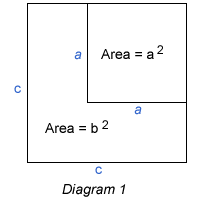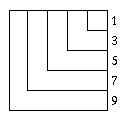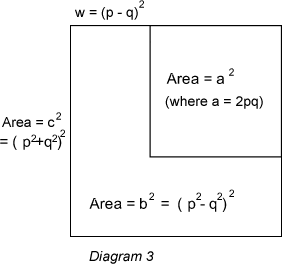# Picturing Pythagorean Triples

##### Age 14 to 18

Published 1998 Revised 2009

 Somewhat surprisingly every Pythagorean triple $( a, b, c)$, where $a, b$ and $c$ are positive integers and $a^2+b^2 = c^2$, can be illustrated by this diagram, in which the L shaped region has area $b^2$, and the areas of the larger and smaller squares are $c^2$ and $a^2$. With this clue you can find some triples for yourself right away. With an L strip of width 1 unit you get the whole class of Pythagorean triples with $a$ and $c$ as consecutive integers, that is $c = a+1.$Diagram 2 This diagram (extended as far as required) illustrates the fact that, for all $n$, the sum of the first $n$ odd numbers gives $n^2$. As we can see from the diagram to the left: \begin{eqnarray}1+3&=&2^2&=&4 \\ 1+3+5 &=& 3^2 &=& 9 \\ 1+3 +5 + 7 &=& 4^2 &=& 16 \\ 1+3+5+7+9 &=& 5^2 &=& 25 \end{eqnarray}

Taking $a= 4$ and $c= 5$, the area of the outer L'' strip is $b^2= 9$ and this gives the first Pythagorean triple $4^2+ 3^2= 5^2$.

Before reading on, can you use more strips of unit width and extend the diagram to find Pythagorean triples with $b^2 = 25, 49, 81, 121$ etc.?

For every odd square number $b^2$, we can pick out a Pythagorean triple.

As the Diagram 2 shows, $c = a + 1$ and hence \begin{eqnarray} b^2 = c^2 - a^2 \\ b^2 = (a+1)^2 - a^2 \\ b^2 = a^2 + 2a +1 -a^2\\ = 2a + 1 \end{eqnarray} The next odd square after $3^2 = 9$ is $5^2 = 25$
Let $$25 = b^2$$
$$25 = 2*12 + 1$$
giving $a$ = 12 and from there the triple $5^2+12^2=13^2$

The next one in this class is $b=7$ giving $a=24 = \frac{(49-1)}{2}$ and $c=25$

Giving the triple $24^2+7^2=25^2$, and so on.

This method gives us infinitely many Pythagorean triples, but what about others where the width of the L strip (the difference between $a$ and $c$) is more than 1?To find other triples with L strips of different widths try this for yourself: choose any two positive integers $p$ and $q$ that have no common factors and with $p > q$; \item calculate $2p q$ and $p^2+q^2$; \item draw two squares with sides $a=2p q$ and $c = p^2 + q^2$ as shown in Diagram 3. The width of the L strip is $w = (p-q)^2$ and its area is $b^2 = (p^2 -q^2)^2$, and if you wish you can check this algebra for yourself. For example, taking $p= 5$ and $q= 2$, the inner square has dimensions $20$ by $20$ and the outer square has dimensions $29$ by $29$, the width of the L strip is $9$, and the area of the L strip is $(25-4)^2=21^2$, giving the Pythagorean triple $(20, 21, 29)$.
The process given by these instructions produces what is called a parameterisation and $p$ and $q$ are called parameters. It is easy to show by simple algebra that, by choosing positive integers $p$ and $q$, with $p> q$, and taking
$$b = p^2-q^2$$
$$a = 2p q$$
$$c = p^2 + q^2$$
we get a Pythagorean triple.

This does not give all triples, for example it does not give ($9,12,15)$, but it does give all triples for which $a, b$ and $c$ have no common factor (primitive' triples). Every other triple can then be found by calculating $( k a, k b, k c)$ for some integer $k$.

These results are proved in the article Pythagorean Triples II .

We shall concentrate on finding all the triples where $a, b$ and $c$ have no common factor. If $p$ and $q$ have a common factor, or if $p$ and $q$ are both odd, then $a, b$ and $c$ will have a common factor.

We only need the cases where one of $p$ and $q$ is even and the other is odd. In such cases the width of the L strip $= c-a=(p-q)^2$ is always an odd square.

The table below (extended in the obvious way) shows all the primitive' Pythagorean triples, and some non-primitive triples as well.

 Pythagorean Triples $c-b=1^2$ ($p=q+1$) $c-b=3^2$ ($p=q+3$) $c-b=5^2$ ($p=q+5$) $c-b=7^2$ ($p=q+7$) $c-b=9^2$ ($p=q+9$) $c-a=2 \times 1^2$ $(q=1)$ 3, 4, 5 15, 8, 17 35, 12, 37 63, 16, 65 99, 20, 101 $c-a=2 \times 2^2$ $(q=2)$ 5, 12, 13 21, 20, 29 45, 28, 53 77, 36, 85 117, 44, 125 $c-a=2 \times 3^2$ $(q=3)$ 7, 24, 25 27, 36, 45 55, 48, 73 91, 60, 109 135, 72, 153 $c-a=2 \times 4^2$ $(q=4)$ 9, 40, 41 33, 56, 65 65, 72, 97 105, 88, 137 153, 104, 185 $c-a=2 \times 5^2$ $(q=5)$ 11, 60, 61 39, 80, 89 75, 100, 125 119, 120, 169 171, 140, 221 $c-a=2 \times 6^2$ $(q=6)$ 13, 84, 85 45, 108, 117 85, 132, 157 133, 156, 205 189, 180, 261 $c-a=2 \times 7^2$ $(q=7)$ 15, 112, 113 51, 140, 149 95, 168, 193 147, 196, 245 207, 224, 305 $c-a=2 \times 8^2$ $(q=8)$ 17, 144, 145 57, 176, 185 105, 208, 233 161, 240, 289 225, 272, 353 $c-a=2 \times 9^2$ $(q=9)$ 19, 180, 181 63, 216, 225 115, 252, 277 175, 288, 337 243, 324, 405

There is a dual illustration of each triple where the smaller square has side $p^2 - q^2$ and the width of the L strip is $2q^2$. In the dual case for $p=5$ and $q =2$ the inner square has dimensions $21$ by $21$ and the width of the L strip is $8$ units giving the triple $(20,21,29)$.

Given a Pythagorean triple $(a, b, c)$ you can always `work back' to find $p$ and $q$, and given $p$ and $q$ you can always calculate $a, b$ and $c$. You could compete with a friend, both choose a value for $p$ and $q$ and compute a Pythagorean triple $(a, b, c)$ which you give to the other person. Then without using calculators, see who can be the first to find the values of $p$ and $q$. You may need to keep $p$ and $q$ small at first to make this easy for yourselves but as you get better you can take bigger values of $p$ and $q$.